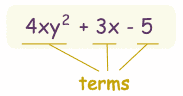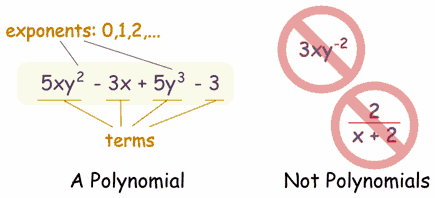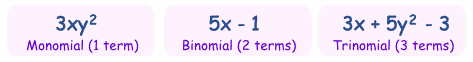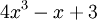## Labels

### NASA Exercise: Survival on the Moon

ACTIVITY

Scenario:
You are a member of a space crew originally scheduled to rendezvous with a
mother ship on the lighted surface of the moon. However, due to mechanical
difficulties, your ship was forced to land at a spot some 200 miles from the
rendezvous point. During reentry and landing, much of the equipment aboard
was damaged and, since survival depends on reaching the mother ship, the
most critical items available must be chosen for the 200-mile trip. Below are
listed the 15 items left intact and undamaged after landing. Your task is to
rank order them in terms of their importance for your crew in allowing them
to reach the rendezvous point. Place the number 1 by the most important item,
the number 2 by the second most important, and so on through number 15 for
the least important.

_______ Box of matches _______
_______ Food concentrate _______
_______ 50 feet of nylon rope _______
_______ Parachute silk _______
_______ Portable heating unit _______
_______ Two .45 caliber pistols _______
_______ One case of dehydrated milk _______
_______ Two 100 lb. tanks of oxygen _______
_______ Stellar map _______
_______ Self-inflating life raft _______
_______ Magnetic compass _______
_______ 20 liters of water _______
_______ Signal flares _______
_______ First aid kit, including injection needle _______

Scoring
For each item, mark the number of points that your score differs from the
NASA ranking, then add up all the points. Disregard plus or minus
differences. The lower the total, the better your score.

0 - 25 excellent
26 - 32 good
33 - 45 average
46 - 55 fair
56 - 70 poor -- suggests use of Earth-bound logic
71 - 112 very poor – you’re one of the casualties of the space program!
... published in the July 1999 issue of the NightTimes

# Polynomials

A polynomial looks like this:example of a polynomial this one has 3 terms

Polynomial comes from poly- (meaning "many") and -nomial (in this case meaning "term") ... so it says "many terms"

A polynomial can have:

 constants (like 3, -20, or ½) variables (like x and y) exponents (like the 2 in y2), but only 0, 1, 2, 3, ... etc are allowed
that can be combined using addition, subtraction, multiplication and division ...

... except ...

 ... not division by a variable (so something like 2/x is right out)
So:

A polynomial can have constants, variables and exponents,
but never division by a variable.

## Polynomial or Not?These are polynomials:

• 3x
• x - 2
• -6y2 - (7/9)x
• 3xyz + 3xy2z - 0,1xz - 200y + 0,5
• 512v5+ 99w5
• 5
(Yes, even "5" is a polynomial, one term is allowed, and it can even be just a constant!)
And these are not polynomials

• 3xy-2 is not, because the exponent is "-2" (exponents can only be 0,1,2,...)
• 2/(x+2) is not, because dividing by a variable is not allowed
• 1/x is not either
• √x is not, because the exponent is "½"
But these are allowed:

• x/2 is allowed, because you can divide by a constant
• also 3x/8 for the same reason
• √2 is allowed, because it is a constant (= 1,4142...etc)

## Monomial, Binomial, Trinomial

There are special names for polynomials with 1, 2 or 3 terms:How do you remember the names? Think cycles!There is also quadrinomial (4 terms) and quintinomial (5 terms),
but those names are not often used.

## Can Have Lots and Lots of Terms

Polynomials can have as many terms as needed, but not an infinite number of terms.

## Variables

Polynomials can have no variable at all

Example: 21 is a polynomial. It has just one term, which is a constant.
Or one variable

Example: x4-2x2+x has three terms, but only one variable (x)
Or two or more variables

Example: xy4-5x2z has two terms, and three variables (x, y and z)

## What is Special About Polynomials?

Because of the strict definition, polynomials are easy to work with.
For example we know that:

So you can do lots of additions and multiplications, and still have a polynomial as the result.

You can also divide polynomials (but the result may not be a polynomial).

## Degree

The degree of a polynomial with only one variable is the largest exponent of that variable.

### Example:The Degree is 3 (the largest exponent of x)
For more complicated cases, read Degree (of an Expression).

## Standard Form

The Standard Form for writing a polynomial is to put the terms with the highest degree first.

### Example: Put this in Standard Form: 3x2 - 7 + 4x3 + x6

The highest degree is 6, so that goes first, then 3, 2 and then the constant last:

x6 + 4x3 + 3x2 - 7